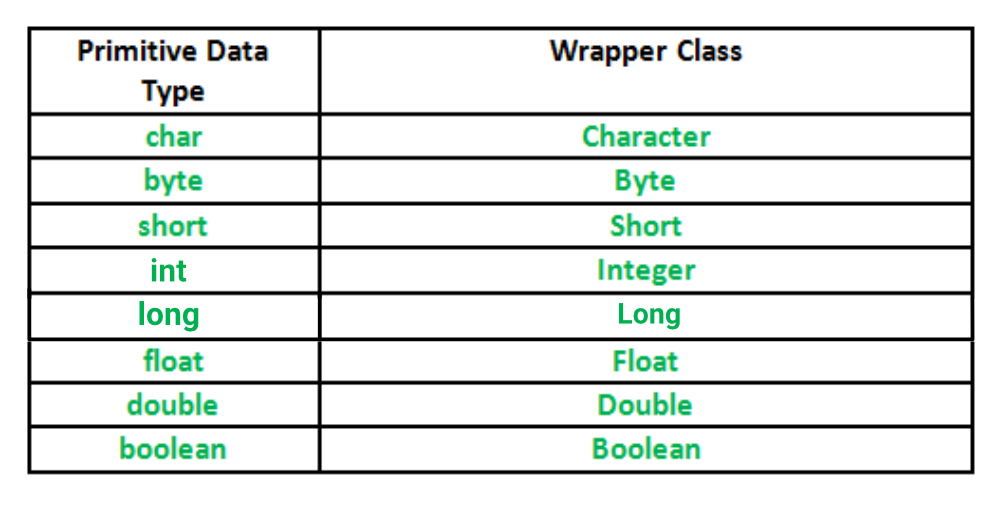Open in App
Not now

# Wrapper Classes in Java

• Difficulty Level : Medium
• Last Updated : 06 Mar, 2023

A Wrapper class in Java is a class whose object wraps or contains primitive data types. When we create an object to a wrapper class, it contains a field and in this field, we can store primitive data types. In other words, we can wrap a primitive value into a wrapper class object. Let’s check on the wrapper classes in java with examples:

## Need of Wrapper Classes

1. They convert primitive data types into objects. Objects are needed if we wish to modify the arguments passed into a method (because primitive types are passed by value).
2. The classes in java.util package handles only objects and hence wrapper classes help in this case also.
3. Data structures in the Collection framework, such as ArrayList and Vector, store only objects (reference types) and not primitive types.
4. An object is needed to support synchronization in multithreading.

Below are given examples of wrapper classes in java with their corresponding Primitive data types in java.

## Primitive Data types and their Corresponding Wrapper class## Autoboxing and Unboxing

### Autoboxing

The automatic conversion of primitive types to the object of their corresponding wrapper classes is known as autoboxing. For example – conversion of int to Integer, long to Long, double to Double, etc.

Example:

## Java

 `// Java program to demonstrate Autoboxing`   `import` `java.util.ArrayList;` `class` `Autoboxing {` `    ``public` `static` `void` `main(String[] args)` `    ``{` `        ``char` `ch = ``'a'``;`   `        ``// Autoboxing- primitive to Character object` `        ``// conversion` `        ``Character a = ch;`   `        ``ArrayList arrayList` `            ``= ``new` `ArrayList();`   `        ``// Autoboxing because ArrayList stores only objects` `        ``arrayList.add(``25``);`   `        ``// printing the values from object` `        ``System.out.println(arrayList.get(``0``));` `    ``}` `}`

Output

`25`

### Unboxing

It is just the reverse process of autoboxing. Automatically converting an object of a wrapper class to its corresponding primitive type is known as unboxing. For example – conversion of Integer to int, Long to long, Double to double, etc.

Example:

## Java

 `// Java program to demonstrate Unboxing` `import` `java.util.ArrayList;`   `class` `Unboxing {` `    ``public` `static` `void` `main(String[] args)` `    ``{` `        ``Character ch = ``'a'``;`   `        ``// unboxing - Character object to primitive` `        ``// conversion` `        ``char` `a = ch;`   `        ``ArrayList arrayList` `            ``= ``new` `ArrayList();` `        ``arrayList.add(``24``);`   `        ``// unboxing because get method returns an Integer` `        ``// object` `        ``int` `num = arrayList.get(``0``);`   `        ``// printing the values from primitive data types` `        ``System.out.println(num);` `    ``}` `}`

Output

`24`

## Java

 `// Java program to demonstrate Wrapping and UnWrapping` `// in Classes` `import` `java.io.*;`   `class` `GFG {` `    ``public` `static` `void` `main(String[] args)` `    ``{` `        ``// byte data type` `        ``byte` `a = ``1``;`   `        ``// wrapping around Byte object` `        ``Byte byteobj = ``new` `Byte(a);`   `        ``// int data type` `        ``int` `b = ``10``;`   `        ``// wrapping around Integer object` `        ``Integer intobj = ``new` `Integer(b);`   `        ``// float data type` `        ``float` `c = ``18``.6f;`   `        ``// wrapping around Float object` `        ``Float floatobj = ``new` `Float(c);`   `        ``// double data type` `        ``double` `d = ``250.5``;`   `        ``// Wrapping around Double object` `        ``Double doubleobj = ``new` `Double(d);`   `        ``// char data type` `        ``char` `e = ``'a'``;`   `        ``// wrapping around Character object` `        ``Character charobj = e;`   `        ``// printing the values from objects` `        ``System.out.println(` `            ``"Values of Wrapper objects (printing as objects)"``);` `        ``System.out.println(``"\nByte object byteobj: "` `                           ``+ byteobj);` `        ``System.out.println(``"\nInteger object intobj: "` `                           ``+ intobj);` `        ``System.out.println(``"\nFloat object floatobj: "` `                           ``+ floatobj);` `        ``System.out.println(``"\nDouble object doubleobj: "` `                           ``+ doubleobj);` `        ``System.out.println(``"\nCharacter object charobj: "` `                           ``+ charobj);`   `        ``// objects to data types (retrieving data types from` `        ``// objects) unwrapping objects to primitive data` `        ``// types` `        ``byte` `bv = byteobj;` `        ``int` `iv = intobj;` `        ``float` `fv = floatobj;` `        ``double` `dv = doubleobj;` `        ``char` `cv = charobj;`   `        ``// printing the values from data types` `        ``System.out.println(``"\nUnwrapped values (printing as data types)"``);` `        ``System.out.println(``"\nbyte value, bv: "` `+ bv);` `        ``System.out.println(``"\nint value, iv: "` `+ iv);` `        ``System.out.println(``"\nfloat value, fv: "` `+ fv);` `        ``System.out.println(``"\ndouble value, dv: "` `+ dv);` `        ``System.out.println(``"\nchar value, cv: "` `+ cv);` `    ``}` `}`

Output

```Values of Wrapper objects (printing as objects)

Byte object byteobj: 1

Integer object intobj: 10

Float object floatobj: 18.6

Double object doubleobj: 250.5

Character object charobj: a

Unwrapped values (printing as data types)

byte value, bv: 1

int value, iv: 10

float value, fv: 18.6

double value, dv: 250.5

char value, cv: a```

My Personal Notes arrow_drop_up
Related Articles